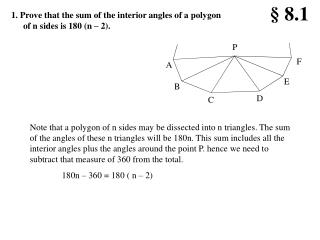Download Presentation1. Prove that the sum of the interior angles of a polygon of n sides is 180 (n – 2).1. Prove that the sum of the interior angles of a polygon of n sides is 180 (n – 2). - PowerPoint PPT Presentation

Download Presentation1. Prove that the sum of the interior angles of a polygon of n sides is 180 (n – 2).
An Image/Link below is provided (as is) to download presentation

Download Policy: Content on the Website is provided to you AS IS for your information and personal use and may not be sold / licensed / shared on other websites without getting consent from its author. While downloading, if for some reason you are not able to download a presentation, the publisher may have deleted the file from their server.

- - - - - - - - - - - - - - - - - - - - - - - - - - - E N D - - - - - - - - - - - - - - - - - - - - - - - - - - -
Presentation Transcript

1. § 8.1 P F A E B D C 1. Prove that the sum of the interior angles of a polygon of n sides is 180 (n – 2). Note that a polygon of n sides may be dissected into n triangles. The sum of the angles of these n triangles will be 180n. This sum includes all the interior angles plus the angles around the point P. hence we need to subtract that measure of 360 from the total. 180n – 360 = 180 ( n – 2)

2. A B E P D C 2. Prove that in a regular polygon of n sides each interior angle has a measure of (180(n – 2))/n From problem 1 we know the sum of the interior angles is 180 (n – 2). And since this is a regular polygon to get the measure of each interior angle we need only divide by n the number of interior angles. Hence each interior angle has a measure of (180 (n – 2))/n. the exterior angle is the supplement of this

3. A B E P D C 3. Prove that in a regular polygon of n sides each exterior angle has a measure of 360/n From problem 1 we know the sum of the interior angles is 180 (n – 2). And since this is a regular polygon to get the measure of each interior angle we need only divide by n the number of interior angles. Hence each interior angle has a measure of (180 (n – 2))/n. The exterior angle is the supplement of this angle and hence has a measure of 180 - (180 (n – 2))/n = 360/n

4. 4. Prove that the sum of the exterior angles of a polygon is 360. Each interior angle and its adjacent exterior angle are supplementary. For each vertex the sum of the exterior and interior angles will be 180 for a total sum of 180n. We know that the sum of the interior angles is 180 (n – 2). The sum of the exterior angles will then be 180 n - 180 (n – 2) = 360 Note this theorem is for all polygons, not just regular polygons..

5. 5. Make a drawing of each of the eight semiregular tessellations

6. 6. Draw an example of a 2-uniform tiling. Don’t use any of those given in class.

7. 7. Will the figure to the right, a bisected regular hexagon, tessellate the plane? Even though each shape is a pentagon, together they form a regular hexagon which tessellates the plane.

8. 8. Research Penrose tiling.

9. 9. Will the figure to the right, a bisected regular hexagon, tessellate the plane? Even though each shape is a pentagon, together they form a regular hexagon which tessellates the plane.